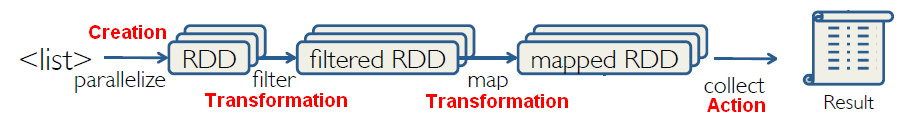# Spark - (Reduce|Aggregate) function

Spark permits to reduce a data set through:

## Reduce

The reduce function of the map reduce framework

````reduce([[..:function|func]])`
```

Reduce is a spark action that aggregates a data set (RDD) element using a function.

That function takes two arguments and returns one.

The function must be (Function | Operator | Map | Mapping | Transformation | Method | Rule | Task | Subroutine) enabled.

reduce can return a single value such as an int.

#### Reduce a List

``````rdd = sc.parallelize([1, 2, 3])
rdd.reduce(lambda a, b: a * b)```
```
``````Value: 6
```
```

#### Reduce a List of Tuple

##### Numeric value

reduceByKey(function|func) return a new distributed dataset of (K, V) pairs where the values for each key are aggregated using the given reduce function func, which must be of type (V,V) → V

``````rdd = sc.parallelize([(1,2), (3,4), (3,6)])
rdd.reduceByKey(lambda a, b: a + b) ```
```
``````RDD: [(1,2), (3,4), (3,6)] → [(1,2), (3,10)]
```
```

If the value is a string, you can use the groupByKey() to reduce it. See below

##### String value

groupByKey() return a new dataset of (K, Iterable

) pairs]] Key-Value Transformations
``````rdd2 = sc.parallelize([(1,'a'), (2,'c'), (1,'b')])
rdd2.groupByKey()```
```
``````RDD: [(1,'a'), (1,'b'), (2,'c')] -> [(1,['a','b']), (2,['c'])]
```
```

Be careful using groupByKey() as it can cause a lot of data movement across the network and create large Iterables at workers

Imagine you have an RDD where you have 1 million pairs that have the key 1. All of the values will have to fit in a single worker if you use group by key. So instead of a group by key, consider using reduced by key or a different key value transformation.

Aggregate Function
• count()
• countApprox
• countApproxDistinct
• sum()
• max
• mean
• meanApprox
• min

Discover MoreSpark - (Reduce|Aggregate) function

Spark permits to reduce a data set through: a reduce function or The reduce function of the map reduce framework Reduce is a spark action that aggregates a data set (RDD) element using a function....Spark - Action

in RDD. Reduce aggregates a data set element using a function. Takeordered and take returns n elements ordered or not Collect returns all of the elements of the RDD as an arraySpark - Group By Key

SeeSpark - Key-Value RDD

Spark supports Key-Value pairs RDD in Python trough a list of tuple. A count of an RDD with tuple will return the number of tuples. A tuple can be seen as a row. Some Key-Value Transformations...